# Splitting the solar radiation in direct and diffuse components; insights and constrains on the clearness-diffuse fraction representation

Splitting the solar radiation in direct and diffuse components; insights and constrains on the clearness-diffuse fraction representation

F. Scarpa*A. Marchitto L.A. Tagliafico

University of Genoa, DIME/TEC, Division of Thermal Energy and Environmental Conditioning, Via All'Opera Pia 15 A, 16145 Genoa, Italy

Corresponding Author Email:
fscarpa@ditec.unige.it
Page:
325-329
|
DOI:
https://doi.org/10.18280/ijht.350213
|
Accepted:
|
Published:
30 June 2017
| Citation

OPEN ACCESS

Abstract:

In many engineering applications, it is mandatory to know separately the solar radiation diffuse and direct components. Examples regard the assessment of the energy potentially exploitable by a system of solar thermal or photovoltaic panels and, in general, all the cases where it is necessary to calculate the radiative solar power collected by a surface. In fact, radiation components will differently project on the surface of interest and will weigh in a different manner, depending on the surface orientation, in the computation of the effective incident radiation. To perform this decomposition starting from data relative to a horizontal plane,two non-dimensional quantities, namely, the diffuse fraction, kd, and the clearness, kt, are usually put in mutual relation by correlating experimental data on a graphical ground rather than using physical considerations. In the present study, some insights are given on the shape of this correlation starting from geometric and physical considerations. It is shown that many results and graphs presented in literature have not physical meaning; rather they are simply artifacts due to geometrical or other constraints. These evidences open the way to a new approach to solar radiation decomposition founded on physical-based correlations.

Keywords:

1. Introduction

Radiation coming from the sun reaches the external atmosphere with a mean power density of about 1367 Wm-2. During its travel to ground, it is in part scattered by air molecules, aerosol and dust, so that only a fraction arrives to us untouched from the original sun direction. This part is called direct component (or direct fraction) of the solar radiation. The scattered part goes toward a twofold destiny; one part is absorbed, captured by particles or molecules, while the other one is scattered and diffused in the atmosphere; it reaches us coming from all the directions of the sky.

The quantitative knowledge of these fractions is of great importance in the assessment of the effective solar radiation collected by a surface. For instance, when estimating the productivity of a solar system , the calculation of the total radiation on tilted surfaces is needed, but this calculation requires the separate knowledge of the direct and diffuse components since they depend in different way on the surface inclination. Similar calculation needs arise when computing the heat load on building facades. Furthermore, the performance of most concentrating solar collector depends mainly on direct radiation .

To summarize, the separate knowledge of direct and diffuse components of solar radiation is important in fields

like solar energy conversion but also in architecture, agriculture, climatology and other areas.

However, mainly due to measurement costs, the values of these separated components are not available in many countries and locations, where only data relative to the radiation on a horizontal plain are available by means of inexpensive instruments like pyranometers. As a consequence, many empirical models were formulated during the past decades to provide reliable prediction of direct and diffuse components of the solar irradiation from readily available data. This decomposition procedure is certainly a difficult task since it is heavily influenced by meteorological conditions, i.e. cloudiness conditions. Moreover, also in case of clear sky, aspects like air humidity, presence of pollutants and other variable factors make solar radiation decomposition a challenging task.

2. Literature Review

The prediction of direct and diffuse components of  the solar radiation is a widely discussed argument among the scientific community. According to various Authors ,, in the literature on the specific subject, two different approaches can be noticed to predict the direct and diffuse (also “beam” and “sky”) components of  solar radiation which are based on more easily measured quantities:

- Parametric models

- Decomposition models

Parametric models are based on accurate information  about atmospheric components and conditions. Very briefly, the beam component is described by multiplying the solar constant, on average 1367 Wm-2, by a series of attenuation factors linked to various physical phenomena. The approach, in synthesis, proceeds by an accurate identification and description of the causes of radiation attenuation and then applies geometric consideration to account for zenith angle and air mass. Finally ground and clouds albedo are introduced to obtain an expression for the diffuse component. An example of such an approach is given by the Iqbal  model. Parametric models are not synthetized in easily applicable formulas, rather, there are complex software tools  and “methods” often based on extensive look-up tables.

Conversely, decomposition models leave the physical based view of the parametric approach and concentrate on a description of the phenomenon based on empirical correlations. Fitting of historical experimental data are made without any reference to a physical justified formulation and physical parameters are introduced a posteriori in the correlation to obtain a better fit. Usually, different locations need different fitting functions.

Examples of decomposition models are from Liu and Jordan , Erbs , and more recently from  and , while a comprehensive review is found in .

In the present study some experimental radiation data presented in the literature, and interpreted under the view point of the decomposition approach, are investigated. The hypothesis is made that some results may derive from misinterpretation of data and that simple geometrical considerations can account for reported behaviors, which are instead accepted, erroneously, as precise solar radiation features. It is shown that various results and graphs presented in literature have not physical meaning; rather they are probably artifacts due to geometrical or other constraints.

3. Clearness and Diffuse Fraction

As said, decomposition methods focus on a simple description of the physical phenomenon, which is often reported as a “sigmoid” curve, which poses in relation the diffuse fraction and the clearness as from the example image of Figure 1. In fact, in such a figure, to quantify and describe the different weights of direct and diffuse fraction, two non- dimensional quantity, namely, the diffuse fraction, kd, and the clearness, kt, defined as follow, are introduced:

$k_{d}=\frac{g_{d}}{g}, k_{t}=\frac{g}{g_{i n}}$     （1）

where g is the incident radiation on a horizontal surface, gd is the diffuse component and gin is the virtual radiation hitting the surface in absence of atmosphere (no attenuation).

These quantities are usually put in mutual relation by using various correlation selected on a ground of best “graphical” fitting rather than on physical considerations.

## 1.png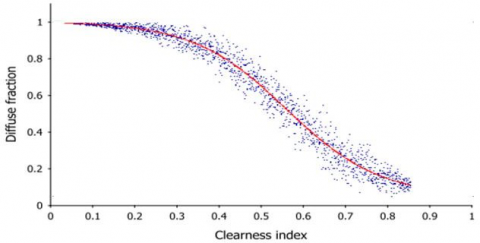Figure 1. Diffuse fraction as a function of the clearness index. Typical behavior

Experimental points on kd-kt plane often spread over a large region since measurement cover usually a long time period, years in some cases (see for instance Figure 4)

Usually the radiation terms appearing in the above definitions are average values with reference to various time periods; typically one minute, one hour or the like. Averaging is “a must” under variable sky conditions, to provide a smoothing to the effect introduced by the presence of moving clouds (cloud–no cloud) but also to filter, and so attenuate, the influence of measurement errors.

Data is in any case recorded for long periods of time and the resulting kd-kt graph often present a pronounced scatter of points due to the variation, within days, weeks etc., of the atmospheric physical condition from the point of view of seasonal effects, chemical pollutant presence, average cloudiness and so on.Raw data are usually integrated over periods of minutes or hours and, due to the experimental origin, they are usually subjected to a preliminary conditioning to eliminate physical inconsistency.

4. Physical Constraints

Let us critically analyze the introduced quantities and their constrains.

In clear sky condition, the direct (beam) radiation, gbh hitting a horizontal plane will be attenuated due to various scattering and absorption. There are numerous concurrent phenomena which contribute to this attenuation. They are described in details for instance in , but it will suffice, at this point, to describe this attenuation by using a single transmission factor t a in the range between 0 and 1. So it  will be

$g_{b h}=g_{i n} \cdot \tau_{a}$     （1）

The global radiation on a horizontal plane is the sum of direct plus diffuse components so that

$g=g_{b h}+g_{d}$     （2）

By substitution in Eq. (1) it follow that

$k_{t}=\frac{g}{g_{i n}}=\frac{g_{i n} \cdot \tau_{a}+g_{d}}{g_{i n}}$     （3）

that is

$k_{t}=\tau_{a}+\frac{g_{d}}{g_{t n}}$     （4）

We here get a first important consideration: the clearness index is the sum of two positive quantities, and that these amounts represent individually a lower bound. Furthermore, the clearness, in the absence of clouds, can never be lower than the ta value, which represents the attenuation coefficient as a function of opacity and of the air thickness traversed by the solar beam.So diagrams, however mediated, declaring completely  clear days and presenting values of kt = 0 or very small, are wrong or contaminated by data collected in cloudy conditions.

In fact the conditions $k_{t}>\tau_{a}$ and $k_{t}>\frac{g_{d}}{g_{i n}}$ should be both true at the same time.

Elaborating on the definition of diffuse fraction, kd, it follows

$k_{d}=\frac{g_{d}}{g}=\frac{g-g_{b h}}{g}=1-\frac{g_{i n}}{g} \tau_{a}$

and so

$k_{d}=1-\frac{\tau_{a}}{k_{t}}$     (5)

Obviously, this relation does not provide an explicit link between clearness and diffuse fraction, as ta and kt are in turn related. It still represents a relation useful to describe a specific constraint.

Suppose, at a certain time of the day, to be under a given sky characterized, for instance, by an attenuation factor ta =0.7. In virtue of Eq.(5), we can safely say that at that moment the diffuse fraction will have to be somewhere on the following curve:

$k_{d}=1-\frac{0.7}{k_{t}}$     (6)

That is on the curve represented in Figure 2.

Always with reference to clear sky conditions, it is worth noting that, speaking about the earth’s atmosphere, the maximum value for ta, that is the minimal attenuation is roughly computed as per [12,13]:

$\tau_{a}=\exp \left(-\delta_{R} \cdot m_{A \min } \cdot T_{L \min }\right)$     (7)

where mAmin is the minimum air mass value occurring with the sun at the zenith, Tmin is a reasonable lower bound for the Linke turbidity, the parameter describing the opacity of air, occurring in case of low presence of pollutant in a very clear and dry day, and dR is a proportionality coefficient.

In  this  condition  we  can  approximatively  put  mAmin  =1T=1.5 and dR=.097 to obtain an upper bound for ta given byta max  @ 0.83. In other words the right down region of figure 1 cannot be reached on the Earth planet , maybe on Mars, since it will be

$k_{d}>1-\frac{\tau_{\mathrm{a} \max }}{k_{t}}$     (8)

Definitely, ta depends on solar elevation since it is a function of the thickness of the traversed atmosphere. The effect is more pronounced at higher latitudes since the sun is usually lower, for instance, at noon.

Anyway, if we consider a medium value latitude such as 45° (Milan, Italy), the max sun elevation at noon in October will be equal to about 30° with a consequent value mAmin @ 2.Setting the turbidity to T=4, a typical urban value (in Milan we can easily find T>5 due to polluted air), the associated transmission factor will be about 0.5. In other words, since ta can assume only values smaller than 0.5, which represent a maximum, the greyed zone of Fig. 3 represents a not reachable region in that month, according to Eq.(8).

## 2.pngFigure 2. Possible values of the diffuse fraction under clear sky condition with an air attenuation factor ta = 0.7.

## 3.png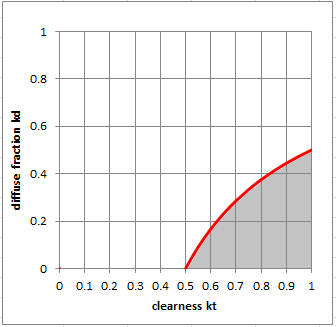Figure 3. Out of reach zone (greyed) in case of ta < 0.5.

5. Experimental Data Interpretation

These considerations have a precise experimental confirmation as clearly shown, for instance, in Figure 4, which reports data collected from the wheatear station of Payern, CH . The figure represents thousands of measures during a year and the effect of weather conditions causes a large spread in the kd-kt plane. In any case, apart from some artifacts, it is evident that there is a zone (bottom right) that is not accessible experimentally.

## 4.png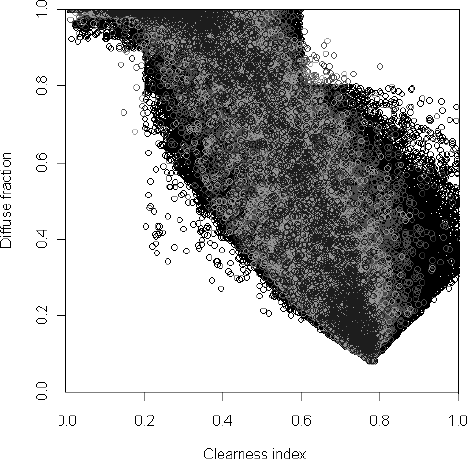Figure 4. Out of reach zone. Experimental evidence .

## 5.png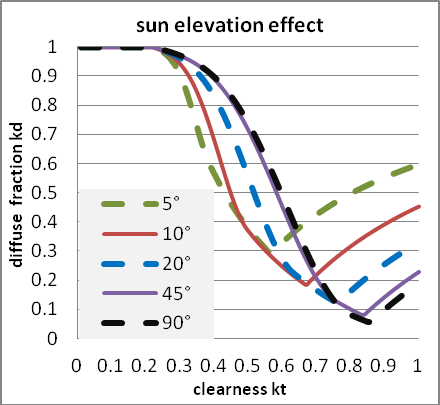Figure 5. Skartveit diffuse fraction model . Influence of solar elevation.

## 6.png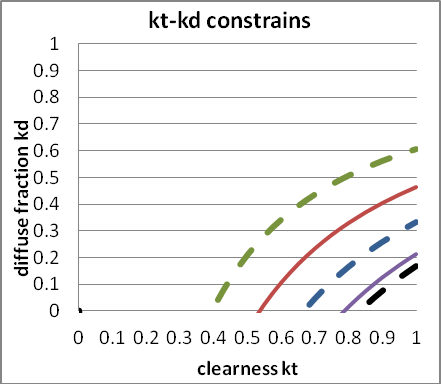Figure 6. Constraint curve from Eq.(5). Same solar elevation angle values of Fig.5

Unfortunately, this was not the interpretation given in the literature. In fact, this kind of experimental data lead various researcher, e.g. Skartveit [15,16] to confuse a physical constraint with a possible functional relationship between clearness and diffuse fraction and suggested some unlikely empirical correlations with strange tails (see Figure 5).

A comparison with Fig. 6 shows that the tails are actually the limits corresponding to the given solar elevations, so some doubts arise about Skartveit diffusion fraction modeling. Constraints on Fig. 6 refer to the same angle values as Fig.5, a turbidity value T=1.5 (a very clear and dry sky, Skartveit elaborates his model mainly from data collected in Norway and he underline the low value of turbidity). The expression for dR, as a function of mA is from  , that is

\begin{aligned} \delta_{\overline{R}}=6.5567+1.7513 \cdot & m-0.1202 \cdot m^{2}+\\ &+0.006 \cdot m_{A}^{3}-0.00013 \cdot m_{A}^{4} \end{aligned}     (9)

while the air mass is expressed, modelling the atmosphere as a simple spherical shell, as

$m_{A}=\sqrt{(r \cos \phi)^{2}+2 r+1}-r \cos \phi$     （10）

with r=708 the ratio between the Earth’s radius and the effective height of the atmosphere.

Some of the classic studies [8, 18], modeled the diffuse fraction as a straight constant in that region.

The true point instead is that the kd-kt sigmoid stops at some point in the right–low part of the diagram due to the described constraints described in this work. Experimental points around that limit are a consequence of the variability of some atmospheric or ground characteristics during the observation period (usually very long). Possible parameters are ground and cloud albedo and various transmission coefficient linked both to beam and diffuse radiation. Finally, the presence of clouds represents the more important stochastic factor in modeling the component of solar radiation; this first-step study is made under the assumption of clear – cloudless sky.

6. Conclusions

In the presented study, some comments are made about solar radiation decomposition models found in literature. It seems that, in some cases, the experimental data have been misinterpreted and that, on these bases, a series of questionable models were developed.

It is possible that better models could emerge from a closer review of the subject, by a synthesis of parametric and decomposition models.

In this study, simple elements derived from physical consideration have clarified some experimental aspects not explained by nonphysical correlations, based only on a “graphic” fitting of raw data.

If physical elements like transmission factors (direct and diffuse), forward and backward scattering characteristics, ground albedo ( also the snow effect), cloud albedo and other elements will be introduced to construct kd-kt correlations based on parametric methods, a greater flexibility will result in the way of the sometime cited, but never reached, “Universal Model”. A model which is capable to adapt itself to every locations and meteorological conditions. A model useful to predict the performance of heating and cooling system for building  or to help the design of large solar fields .

Acknowledgment

Authors want to acknowledge the support of the Italian Ministry of Education, University and Research (PRIN 2015 project n. 2015M8S2_008PA).

Nomenclature
 g incidend solar radiation , Wm-2 kd kt diffuse fraction clearness index mA r air mass (Earth’s radius)/(atmosphere height) ratio T turbidity Greek symbols dR proportional coefficient Eq.(7) j solar elevation angle t transmission coefficient Subscripts a air bh direct (beam)-horizontal plane d diffuse in extraterrestrial on h-plane max maximum min minimum
References

 Mazzeo D., Matera N., Bevilacqua P., Arcuri N.(2015). Energy and economic analysis of solar photovoltaic plants located at the University of Calabria, Int. J. Heat & Tech., Vol. 33, No. 4, pp. 41-50. DOI: 10.18280/ijht.330406

 Duffie J.A., Beckman W.A. (2006). Solar engineering of Thermal Processes, 3rd ed., John Wiley & Sons Inc.,New York.

 Ahmad M.J., Tiwari G.N. (2011). Solar radiation models – a review, Int. J. Energy Res., Vol. 35, pp.271-290. DOI: 10.1002/er.1690

 Bortolini M., Gamberi M., Graziani A., Manzini R., Mora C. (2013). Multi-location model for the estimation of the horizontal daily diffuse fraction of solar radiation in Europe, Energ. Conv. Manag., Vol. 67, pp. 208-216. DOI:10.1016/j.enconman.2012.11.008

 Wong L.T., Chow W.K. (2001). Solar radiation model,Appl. Energy, Vol. 69, pp. 191-224. DOI:10.1016/S0306-2619(01)00012-5

 Maxwell E.L. (1987). A quasi-physical model for converting hourly global horizontal to direct normal insolation, Solar Energy Research Institute, Golden,CO, Tech. Rep. No. SERI/TR-215-3087.

 Liu B., Jordan R. (1960). The interrelationship and characteristic distribution of direct, diffuse and total solar radiation, Sol. Energy, Vol. 4, No. 3, pp. 1-19.DOI: 10.1016/0038-092X(60)90062-1

 Erbs D.G., Klein S.A., Duffie J.A. (1982). Estimation of the diffuse radiation fraction for hourly, daily and monthly-average global radiation, Sol. Energy, Vol. 28.No. 4, pp. 293-302, 1982. DOI: 10.1016/0038-092X(82)90302-4.

 Bakirci K. (2015). Models for the estimation of diffuse solar radiation for typical cities in Turkey, Energy, Vol.82, pp. 827-838. DOI: 10.1016/j.energy.2015.01.093

 Despotovic M., Nedic V., Despotovic D., Cvetanovic S.(2016). Evaluation of empirical models for predicting monthly mean horizontal diffuse solar radiation, Renew. Sust. Energ. Rev., Vol. 56, pp. 246-260. DOI:10.1016/j.rser.2015.11.058

 Kasten F. (1996). The linke turbidity factor based on improved values of the integral Rayleigh optical thickness, Sol. Energy, Vol. 56, No. 3, pp. 239-244.DOI: 10.1016/0038-092X(95)00114-7

 Chaâbane M., Masmoudi M., Medhioub K. (2004).Determination of Linke turbidity factor from solar radiation measurement in northern Tunisia, Renew. Energy, Vol. 29, No. 13, pp. 2065-2076. DOI:10.1016/j.renene.2004.03.002

 Lanini F., (2010). Division of global radiation into direct and diffuse radiation, Master’s thesis, University of Bern, Bern, Switzerland.

 Skartveit A., Olseth J.A. (1987). A model for the diffuse fraction of hourly global radiation, Sol. Energy, Vol. 38,No. 4, pp. 271-274. DOI: 10.1016/0038-092X(87)90049-1

 Skartveit A., Olseth J.A., Tuft M.E. (1998). An hourly diffuse fraction model with correction for variability and surface albedo, Sol. Energy, Vol. 63, No. 3, pp.173-183. DOI: 10.1016/S0038-092X(98)00067-X

 Louche A., Peri G., Iabal M. (1986). An analysis of the Linke turbidity factor, Sol. Energy, Vol. 37, No. 6, pp.393-396. DOI: 10.1016/0038-092X(86)90028-9

 Orgill J.F., Hollands K.G.T. (1977). Correlation equation for hourly diffuse radiation on a horizontal surface, Sol. Energy, Vol. 19, No. 4, pp. 357-359. DOI:10.1016/0038-092X(77)90006-8

 Cascetta F., Cirillo L., della Corte A., Nardini S. (2017).Comparison between different solar cooling thermally driven system solutions for an office building in Mediterranean Area, Int J Heat & Tech, Vol. 35, No. 1,pp. 130-138. DOI: 10.18280/ijht.350118

 Rovense F., Amelio M., Ferraro V., Scornaienchi N.M.(2016). Analysis of a concentrating solar power tower operating with a closed joule Brayton cycle and thermal storage, Int J Heat & Tech, Vol. 34, No. 3, pp. 485-490.DOI: 10.18280/ijht.340319